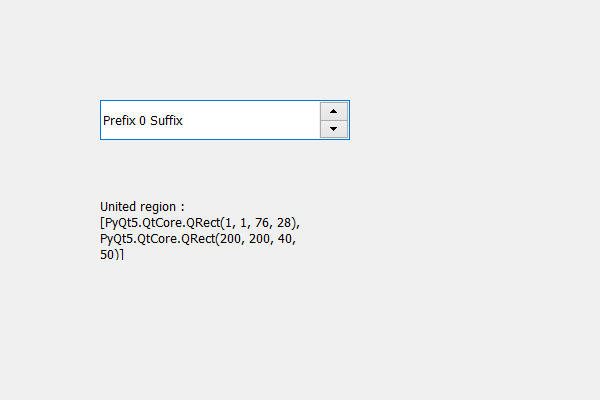# PyQt5 QSpinBox – Get the united region with Children region

• Last Updated : 19 May, 2020

In this article we will see how we can get the united with the children region of spin box and another region, children region holds the combined region occupied by the spin box’s children. In order to get the children region we use `childrenRegion` method. United region will be the union of the children region and the children region

In order to do this we use united method with the children region object of the spin box

Syntax : children_region.united(rectangle)

Argument : It takes QRect as argument also it can take QRegion

Return : It returns None

Below is the implementation

 `# importing libraries``from` `PyQt5.QtWidgets ``import` `*` `from` `PyQt5 ``import` `QtCore, QtGui``from` `PyQt5.QtGui ``import` `*` `from` `PyQt5.QtCore ``import` `*` `import` `sys`` ` ` ` `class` `Window(QMainWindow):`` ` `    ``def` `__init__(``self``):``        ``super``().__init__()`` ` `        ``# setting title``        ``self``.setWindowTitle(``"Python "``)`` ` `        ``# setting geometry``        ``self``.setGeometry(``100``, ``100``, ``600``, ``400``)`` ` `        ``# calling method``        ``self``.UiComponents()`` ` `        ``# showing all the widgets``        ``self``.show()`` ` `        ``# method for widgets`` ` `    ``def` `UiComponents(``self``):``        ``# creating spin box``        ``self``.spin ``=` `QSpinBox(``self``)`` ` `        ``# setting geometry to spin box``        ``self``.spin.setGeometry(``100``, ``100``, ``250``, ``40``)`` ` `        ``# setting range to the spin box``        ``self``.spin.setRange(``0``, ``999999``)`` ` `        ``# setting prefix to spin``        ``self``.spin.setPrefix(``"Prefix "``)`` ` `        ``# setting suffix to spin``        ``self``.spin.setSuffix(``" Suffix"``)`` ` `        ``# getting the children region``        ``children_region ``=` `self``.spin.childrenRegion()`` ` `        ``# creating a label``        ``label ``=` `QLabel(``self``)``        ``label.setWordWrap(``True``)`` ` `        ``# setting geometry to the label``        ``label.setGeometry(``100``, ``200``, ``200``, ``60``)`` ` `        ``# QRect``        ``rectangle ``=` `QRect(``200``, ``200``, ``40``, ``50``)`` ` `        ``# getting united region with the children region``        ``children_region ``=` `children_region.united(rectangle)`` ` `        ``# setting text to the label``        ``label.setText(``"United region : "` `+` `str``(children_region.rects()))`` ` ` ` `# create pyqt5 app``App ``=` `QApplication(sys.argv)`` ` `# create the instance of our Window``window ``=` `Window()``window.spin.setFocus()``# start the app``sys.exit(App.``exec``())`

Output :My Personal Notes arrow_drop_up